Mitchell, P. (2019). "Development of an air drying index for lumber," BioRes. 14(3), 6657-6665.

#### Abstract

Lumber is often air dried in an air drying yard prior to being placed in a dry kiln for final moisture content removal. The rate of the air drying process is dependent on local weather conditions and wood moisture content. Introduced more than 30 years ago, the Drying Index is a method to estimate the relative drying time using the difference in vapor pressures between the dry bulb and wet bulb temperatures. This paper extends its application and describes the development and use of an Air Drying Index to estimate the relative potential of a geographic location to air dry lumber based on the average monthly climate conditions for that location. The monthly Air Drying Index for 252 US locations is presented, and the annual mean for each location was used to develop an air drying map.

Development of an Air Drying Index for Lumber

Philip H. Mitchell *

Lumber is often air dried in an air drying yard prior to being placed in a dry kiln for final moisture content removal. The rate of the air drying process is dependent on local weather conditions and wood moisture content. Introduced more than 30 years ago, the Drying Index is a method to estimate the relative drying time using the difference in vapor pressures between the dry bulb and wet bulb temperatures. This paper extends its application and describes the development and use of an Air Drying Index to estimate the relative potential of a geographic location to air dry lumber based on the average monthly climate conditions for that location. The monthly Air Drying Index for 252 US locations is presented, and the annual mean for each location was used to develop an air drying map.

Keywords: Wood moisture content; Equilibrium moisture content; Lumber drying

Contact information: Department of Forest Biomaterials, North Carolina State University, Campus Box 8003, Raleigh, NC 27695-8003 USA; *Corresponding author: phil_mitchell@ncsu.edu

INTRODUCTION

The question of precisely how much time it will take to dry lumber can be difficult to answer because of all the variables involved. In an environment maintained at a constant relative humidity and temperature, wood will reach a moisture content that is in equilibrium with the surrounding air. Temperature and relative humidity determine the equilibrium moisture content (EMC) condition, and the moisture content of wood in a given environment will approach that EMC. Knowing the average EMC of an environment or a geographic location can provide an indication of how fast lumber might dry if stacked on an air drying yard.

Lewis (1985) introduced the Drying Index as a method that can be used to estimate the relative drying time. This method states that the “speed of drying is related to the vapor pressure deficit” between the dry bulb and wet bulb temperatures and that the deficit value can be used to compare the relative drying rates between different EMC conditions.

Two recent publications from NC State University Wood Products Extension have determined the average monthly EMC’s based on climate data for 252 locations in the US (Mitchell 2016), and used that geographic climate data to estimate the length of time required to air dry 4/4 (1-inch thick) lumber to a target moisture content for red oak, yellow poplar, and sugar maple (Mitchell 2018a).

Maps illustrating zones having the same number of “good air drying months” have been published (Forest Products Laboratory 1999; Denig et al. 2000). The zone boundaries are largely based on the average cumulative “growing degree days” from March 1 to mid-October for 1971 to 1975 (Denig et al 2000). These published maps are based on older and limited data, and are only indirectly related to the air drying of lumber.

The purpose of this paper is to describe the development and use of an Air Drying Index (ADI) to estimate the relative potential of a US geographic location to air dry lumber based on the average monthly climate conditions for that location, and then to use that information to develop an air drying map.

EXPERIMENTAL

Drying Index as a Measure of the Speed of Drying

Figure 1 presents Lewis’ Drying Index as a function of the dry bulb temperature and wet bulb depression. For two different sets of drying conditions (as described by dry bulb temperature and wet bulb depression), the ratio of the two drying indices provides a relative measure of the drying speed between the two conditions.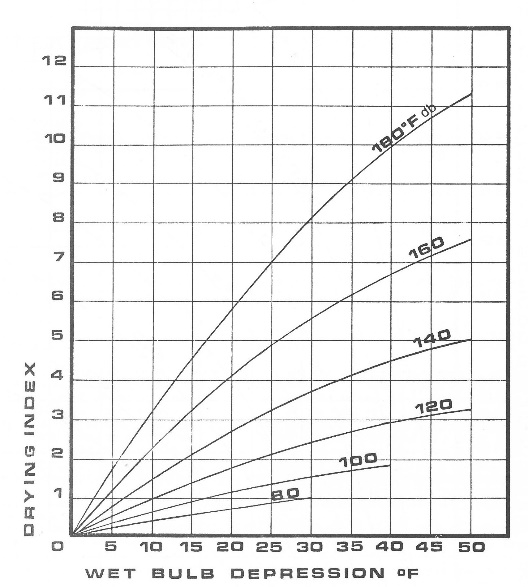Fig. 1. Drying index as a function of dry bulb temperature and wet bulb depression (Lewis 1985)

A mathematical relationship that calculates the drying index from the dry bulb temperature and wet bulb depression was derived from Fig. 1 and is presented as Eq. 1. This equation allows the Drying Index to be calculated based on knowledge of the dry bulb and wet bulb temperatures. Although using this equation to calculate the Drying Index for most air drying conditions does extrapolate beyond the conditions shown in Fig. 1 that were used to derive it, the calculated Drying Index does provide a method to compare drying conditions,

Drying Index = 0.00229 DBT + 0.04038 (po-pw) (1)

where DBT is dry bulb temperature (°F), po is the vapor pressure at the dry bulb temperature (mm Hg), and pw is the vapor pressure at the wet bulb temperature (mm Hg).

Use of Climate Normals to Calculate Air Drying Index (ADI)

In a recent paper, 30-year Climate Normals covering the period from 1981 to 2010 for 252 US locations (NCDC 2016) were used to calculate the EMC based on the average monthly temperature and relative humidity for each location (Mitchell 2016). For the purposes of this paper, the Climate Normals for each location were used along with Eq. 1 to describe an average Air Drying Index (ADI) for each month at each location. Although this application of Eq. 1 was used at temperatures that extrapolate beyond Lewis’ paper, the concept of relating vapor pressure differential to the relative drying rate is preserved.

Estimating Average Daily Moisture Content Loss During Air Drying

To match the ADI developed from Climate Normals as described above, an estimate of moisture loss during air drying based on the same Climate Normals was required. For the air drying of 4/4 red oak, Denig and Wengert (1982) presented the following equation to estimate the daily percent moisture content (%MC) loss based on initial %MC, temperature, and percent relative humidity,

Daily %MC Loss = 0.27 + 0.00071 (MC2) + 0.025 (DBT) – 0.031 (RH) (2)

where MC is the daily initial moisture content (percent), DBT is the average monthly dry bulb temperature (°F), and RH is the average monthly relative humidity (percent). Equation 2 was one of the air drying models used in developing the Excel spreadsheet “Air Drying Estimator” (Mitchell 2018b).

As illustrated by Eq. 2, the initial %MC exerts considerable influence on the Daily %MC Loss calculated. This agrees with the fact that the initial drying rate of green lumber is much higher than the rate during the later stages of drying when the diffusion of bound water from the interior to the wood surface limits the drying rate. Hence, daily drying rates were calculated based on this equation for lumber starting at 80% and 40% average moisture contents as a function of mean monthly temperature and relative humidity. Separately, for these two moisture content groups, the ADI and Daily %MC Loss were paired for each month and location (with equal Climate Normals for each month and location). Graphs of the results are presented in Fig. 2.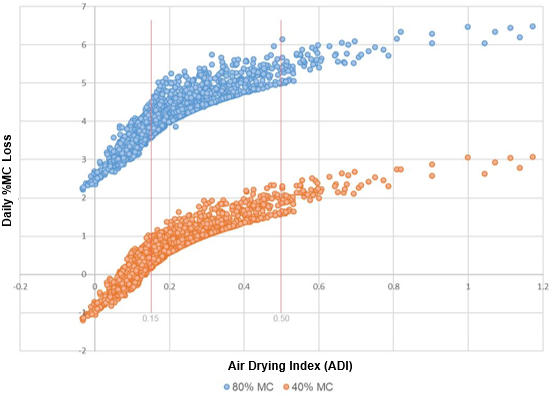Fig. 2. Average daily %MC loss versus monthly air drying index (ADI) for lumber having an initial %MC of either 80% or 40% MC.

In summary, Climate Normals were used to calculate a monthly Air Drying Index using Eq. 1, and to calculate the Daily %MC Loss using Eq. 2. The relationship between the resulting calculated ADI and the Daily %MC Loss having the same Climate Normal is presented in Fig. 2. The goal is to use the ADI value to determine whether, on average, “good” air drying conditions exist for a given month and location.

RESULTS AND DISCUSSION

“Good” Air Drying Conditions

“Good” air drying is described (Denig et al. 2000) as being able to dry 4/4 oak to 25% MC in 90 days, with the “best” air drying only taking 60 days. Assuming an initial moisture content of 80%, “good” air drying removes an average 0.61% MC per day, while the “best” air drying removes 0.92% MC per day. For the purposes of this paper, “good” air drying is defined as drying 0.61% MC (or more) per day. Of course, much higher drying rates may be excessive for the species being dried and may cause drying degrade. Denig et al. (2000) presents the safe drying rates (%MC loss per day) for selected species that will result in high quality dried lumber. Exceeding this rate increases the risk of quality loss, while drying at rates considerably below the safe rate can increase the risk of staining.

Using ADI to Determine “Good” Monthly Air Drying Conditions

Several points can be realized by examining Fig. 2. First, across the range of ADI from 0.15 to 0.5 (67% of the data points), the Daily %MC Loss for lumber at 80% MC is about 3 to 10 times higher compared to lumber at 40% MC (see Table 1). This means that even when climate conditions are cold and damp (resulting in a relatively low ADI), daily moisture loss is relatively high for very green lumber. This also means that lower moisture content lumber will be the limiting factor in establishing a “good” air drying month (drying rate of 0.61% MC per day or more). Secondly, increasing the ADI from 0.15 to 0.5 has a much larger relative effect on the Daily %MC Loss for lumber at 40% MC compared to 80% MC. As can be seen in Table 1, an increase in the ADI from 0.15 to 0.5 resulted in a corresponding increase in the Daily %MC Loss by a factor of 4.6 for the drier lumber, compared to an increase by a factor of only 1.4 for the wetter lumber.

Table 1. Comparison of the Daily %MC Loss* for Selected ADI’s that Range from 0.15 to 0.5 for Lumber Moisture Contents of 40% and 80%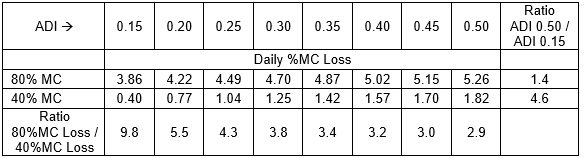* The Daily %MC Loss was calculated by the following equations:

For 80% MC: e(Avg.Daily %MC Loss) = 414.13 ADI – 14.689 R2 = 0.78 (3)

For 40% MC: e(Avg.Daily %MC Loss) = 13.316 ADI – 0.513 R2 = 0.83 (4)

Hence in determining what is a “good” air drying month based on monthly average ADI, one only needs to focus on the lower moisture content lumber group, since the green lumber dries quite well across the ADI range (all months). Figure 3 graphically presents the relationship between e(Daily %MC Loss) and the monthly ADI for the lumber at 40% MC. Also shown in Fig. 3 is the linear regression line for all e(Daily %MC Loss) versus monthly ADI for the lumber at 40% MC. Additionally, Fig. 3 depicts the linear relationship illustrated by the red line drawn along the lowest points which represents the minimum Average Daily %MC Loss for that particular Monthly Air Drying Index, and thereby defines the slowest air drying days for a given ADI. This red line is the minimum Average Daily MC% Loss exhibited by the drier (40% MC) lumber and is described by the equation: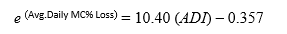(5)

Rearranging Equation 5 to calculate the ADI: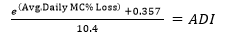(6)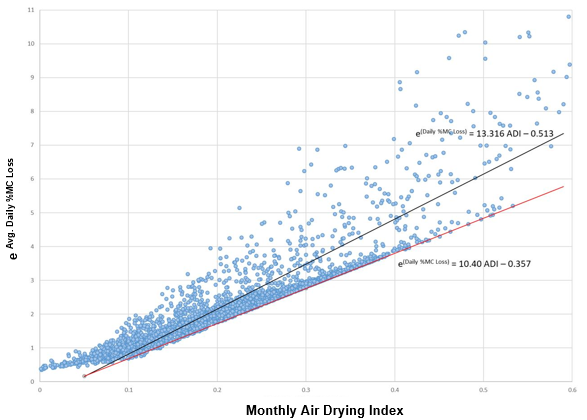Fig. 3. The graph of eAverage Daily %MC Loss versus Monthly Air Drying Index (ADI) for lumber having an initial MC of 40% MC. The black line is the regression line for all data. The red line drawn along the lowest points represents the lowest or minimum Average Daily %MC Loss for that particular value of the Monthly Air Drying Index.

Using the previously defined “good” air drying rate as 0.61% MC loss per day and solving Eq. 6 for ADI results in an ADI of 0.2113. For each month and location, the Air Drying Index (ADI) calculated using Eq. 1 was compared to an ADI of 0.2113. If the ADI was equal to or greater than 0.2113 for the month, that month was considered to be a “good” air drying month. For each location, the total number of “good” or effective air drying months was summed and is presented in Table 2. Keep in mind that in this context, “good” air drying considers only the rate of moisture removal and does not consider drying quality. (An expanded table which shows monthly ADI for 252 US locations is available at: https://go.ncsu.edu/adi-table.)

Creating an Air Drying Map Based on the Air Drying Index

The graphical representation of the Air Drying Index as a map is shown in Fig. 4. The map was created using ArcGIS Pro software from Esri.  The Air Dry Index data for the US locations were interpolated using the Natural Neighbor method, producing a final raster with a cell size of 500 square meters.  From the raster, contour lines were generated at 0.5 intervals between Air Dry Index numbers (0.5, 1.5, 2.5, etc.) in order to clearly illustrate index value changes across the country, including Alaska.